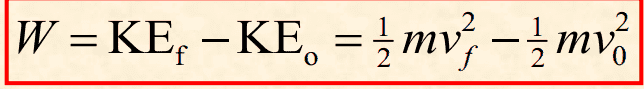# Object is accelerated from rest , find KE

discosucks

## Homework Statement

An object is accelerated from rest by a force of 32 N over a distance of 18 m. Calculate the
kinetic energy of the object after 18 m

## Homework Equations

KE = 0.5 • m • v^2[/B]

## The Attempt at a Solution

I cant work out where ill be able to get the mass or the velocity for this one?

Mentor
I cant work out where ill be able to get the mass or the velocity for this one?
Hint: Maybe you won't need those quantities. Any other principles you know that have to do with energy?

Maged Saeed
I cant work out where ill be able to get the mass or the velocity for this one?

You can work them out if you wish , if we can put 'a' in term of 'm' [From the given force] , we can find the final velocity using the equations of motion with constant acceleration [the acceleration is constant because the force is constant] .

But this method is quite long .However, as Mr @Doc Al said, there is a shorter method that has to do with work and energy , could you figure it out?

:)

discosucks
ummm

well the force by the distance will give me the work done

32 x 18 = 576J

Im almost sure there is a relationship between work and KE but i cant work out what•Maged Saeed
Maged Saeed
ummm

well the force by the distance will give me the work done

32 x 18 = 576J

Im almost sure there is a relationship between work and KE but i cant work out whatWell, have you studied Work-Kinetic energy theorem ?

discosucks
Yes but im not sure i totally understand what to do when with the formula we haveMentor
That's the formula you need! What does it say? (In words.)

discosucks
If im totally honest Im not sure what KEf and KEo stand for .

Maged Saeed
If im totally honest Im not sure what KEf and KEo stand for .

Work-Kinetic energy says :
"For a particle , a change in the kinetic energy equals the net work done on the particle "

In the formula you give :

KEf stands for final kinetic energy of the object.
KE0 stands for initial kinetic energy of the object.

Maged Saeed
I Hope you get the point now ,,.

discosucks
Ah so the work done is the same as the KE !

I know the work done is 576J so thats the same as the KE!

•Maged Saeed
Maged Saeed
The work done is equal to the value of KEf - KE0 But KE0 is zero since the object has started from the rest [the velocity is zero] so the final kinetic energy is equal to the work done by the object..

:)

discosucks
Oh perfect i actually over looked that! thaks again for your help!

•Maged Saeed